Open in App
Not now

# Seaborn Kdeplot – A Comprehensive Guide

• Last Updated : 17 Nov, 2022

Kernel Density Estimate (KDE) Plot and Kdeplot allows us to estimate the probability density function of the continuous or non-parametric from our data set curve in one or more dimensions it means we can create plot a single graph for multiple samples which helps in more efficient data visualization.

In order to use the Seaborn module, we need to install the module using the below command:

`pip install seaborn`

Syntax: seaborn.kdeplot(x=None, *, y=None, vertical=False, palette=None, **kwargs)

Parameters:

x, y : vectors or keys in data

vertical : boolean (True or False)

data : pandas.DataFrame, numpy.ndarray, mapping, or sequence

We learn the usage of some parameters through some specific examples:

First import the corresponding library

## Python3

 `import` `pandas as pd` `import` `seaborn as sb` `import` `numpy as np` `from` `matplotlib ``import` `pyplot as plt` `%``matplotlib inline`

### Draw a simple one-dimensional kde image:

Let’s see the Kde of our variable x-axis and y-axis, so let pass the x variable into the kdeplot() methods.

## Python3

 `# data x and y axis for seaborn` `x``=` `np.random.randn(``200``)` `y ``=` `np.random.randn(``200``)`   `# Kde for x var` `sns.kdeplot(x)`

Output: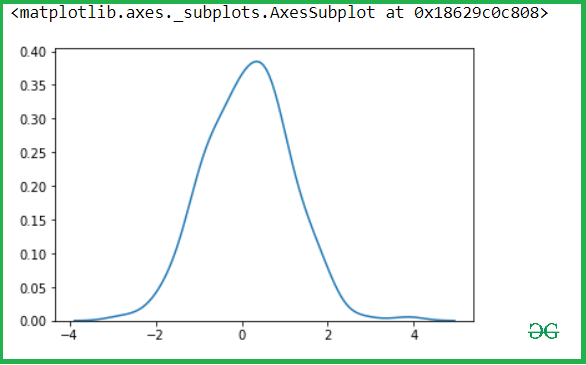Then after check for y-axis.

## Python3

 `sns.kdeplot(y)`

Output: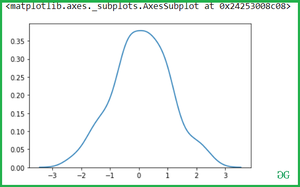### Use Shade to fill the area covered by curve:

We can highlight the plot using shade to the area covered by the curve. If True, shadow processing is performed in the area below the kde curve, and color controls the color of the curve and shadow

## Python3

 `sns.kdeplot(x, shade ``=` `True``)`

Output: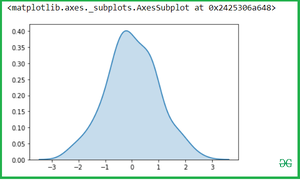You can change the Shade color with color attributes:

## Python3

 `sns.kdeplot(x, shade ``=` `True` `, color ``=` `"Green"``)`

Output: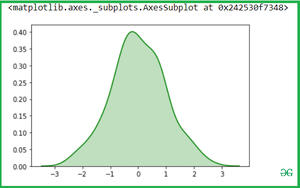## Python3

 `sns.kdeplot(x, vertical ``=` `True``)`

Output: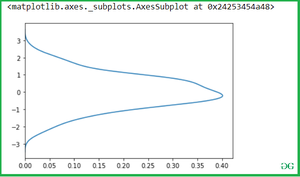### Bivariate Kdeplot for two variables:

Simple pass the two variables into the seaborn.kdeplot() methods.

## Python3

 `sns.kdeplot(x,y)`

Output: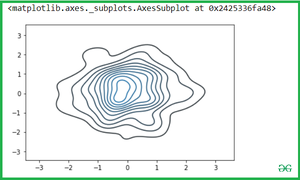## Python3

 `sns.kdeplot(x,y, shade ``=` `True``)`

Output: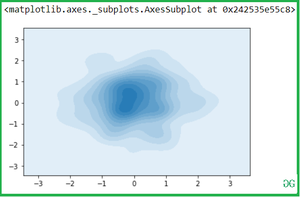Now you can change the color with cmap attributes:

## Python3

 `sns.kdeplot(x,y, cmap ``=` `"winter_r"``)`

Output: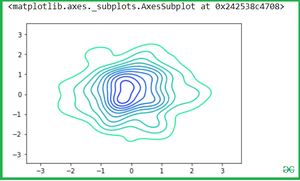Use of Cbar: If True, add a colorbar to annotate the color mapping in a bivariate plot. Note: Does not currently support plots with a hue variable well.

## Python3

 `sns.kdeplot(x, y, shade``=``True``, cbar``=``True``)`

Output: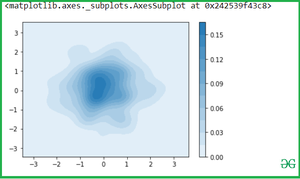Let see the example with Iris Dataset which is plot distributions for each column of a wide-form dataset:

Iris data set consists of 3 different types of irises’ (Setosa, Versicolour, and Virginica) petal and sepal length, stored in a 150×4 numpy.ndarray

## Python3

 `iris ``=` `sns.load_dataset(``'iris'``)` `iris`

Output: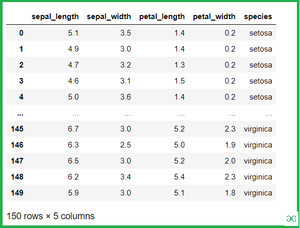### Bivariate Kdeplot for two variables of iris:

Once we have species set then if we want to simply calculate the petal_length and petal_width then Simple pass the two variables(Setosa and virginica ) into the seaborn.kdeplot() methods.

## Python3

 `setosa ``=` `iris.loc[iris.species``=``=``"setosa"``]` `virginica ``=` `iris.loc[iris.species ``=``=` `"virginica"``]` `sns.kdeplot(setosa.petal_length, setosa.petal_width)`

Output: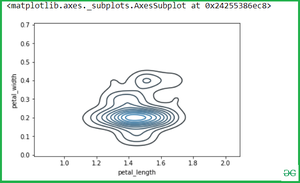See another example if we want to calculate another variable attribute which is sepal_width and sepal_length.

## Python3

 `sns.kdeplot(setosa.sepal_width, setosa.sepal_length)`

Output: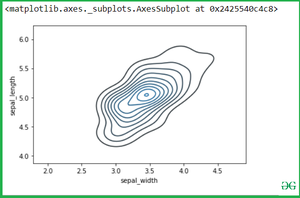If we pass the two separate Kdeplot with different variable:

## Python3

 `sns.kdeplot(setosa.petal_length, setosa.petal_width)` `sns.kdeplot(virginica.petal_length, virginica.petal_width)`

Output: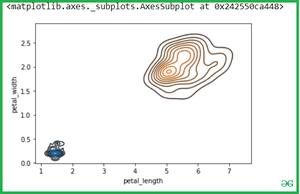My Personal Notes arrow_drop_up
Related Articles